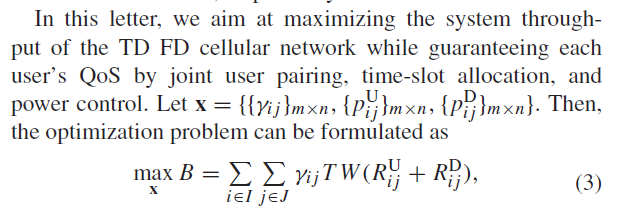# How to implement x1* log(1+x2)

I want to do sth like this:

``````cvx_begin
variables x(2,2,3)
x1=x(:,:,1);
x2=x(:,:,2);
x3=x(:,:,3);
for i=1:2
for j=1:2
y1=x1( i , j )*log2(1+x2( i , j )+x3( i , j ))
end
end
........
maximise (obj)  _% I will use y1 in the obj_
subject to
x(:)>=0
sum(sum(x1)) <= 1
........
``````

I tried log2(1+x2( i , j )+x3( i , j )), it’s permitted,obviously.
I believe y1 is concave but it is Invalid. with message:

Error using .* (line 53)
Disciplined convex programming error:
Invalid operation: {concave} * {real affine}

how can I express this,
I find “rel_entr” in other’s questions but there is only one description in the guide:
† rel_entr(x)
** Scalar relative entropy; rel_entr(x,y)=x.log(x/y). Convex.*
I do not know if this can solve my problem.

Another test i tried like this

``````variables x
variables y
maximize (x*y)
subject to
x<=2
y<=2
x>=0
y>=0
``````

the answer is 4,obviously. but I failed.

By the way,my last question is stupid and i want to delete it. I cann’t find the button of delete .It would be a good idea to edit it to a new quesetion but I’m afriad you will miss it.

x1*log(1+x2) is indefinite, i.e, neither convex nor concave, so there is no reformulation which CVX will accept.

Your topic “Does it mean that the constraint is not enough?” has now been deleted. Hopefully that is the one you wanted to be deleted.

I’m sorry, I do not know clearly about what does “indefinite” mean.I have constraint to make sure the function is increasing in the definition domain.

I’ve tried much.like how can I maximize x*y in cvx?it is Invalid…i can’t understand why.
and can’t i reform the function with rel_entr(x),i don’t find much about how to use it

I’m tying to implement a paper
And the variables and Objective function is:It says like this:

For simplicity,I set rij as x1,Pu as x2.
As this paper says it is concave.however you tell me that is indefinite,do you mean I have missed some of the conditions.or you mean that such function like x1*log2(1+x2+x3) should not be concave
The paper I have saw about d.c. function all use CVX.I have done one before but stuck this time.
I would appreciate it if you could help me

I told you `x1*log(1+x2)` is indefinite, which means that its Hessian with respect to x1 and x2 has both positive and negative eigenvalues, and therefore the expression is neither convex nor concave. However, I don’t think that’s what’s being done in the paper extract you just provided. it is critical that you pay attention to which symbols are optimization variables (decision variables , i.e., what would be CVX variables) vs. which are input data to the optimization problem.

In each term in the difference expression, there is a term which is of the form addressed in Writing a constraint in DCP complient format and can be implemented using rel_entr. . It is then up to you to handle the difference of convex (or concave) functions aspect to implement an algorithm in CVX.